# Determinant of a Matrix

The determinant of a matrix is a special number that can be calculated from a square matrix.

A Matrix is an array of numbers: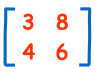A Matrix
(This one has 2 Rows and 2 Columns)

The determinant of that matrix is (calculations are explained later):

3×6 − 8×4 = 18 − 32 = −14

## What is it for?

The determinant helps us find the inverse of a matrix, tells us things about the matrix that are useful in systems of linear equations, calculus and more.

## Symbol

The symbol for determinant is two vertical lines either side.

Example:

|A| means the determinant of the matrix A

(Exactly the same symbol as absolute value.)

## Calculating the Determinant

First of all the matrix must be square (i.e. have the same number of rows as columns). Then it is just basic arithmetic. Here is how:

## For a 2×2 Matrix

For a 2×2 matrix (2 rows and 2 columns):The determinant is:

"The determinant of A equals a times d minus b times c"

 It is easy to remember when you think of a cross: Blue is positive (+ad), Red is negative (−bc)### Example:|B|= 4×8 − 6×3
= 32 − 18
= 14

## For a 3×3 Matrix

For a 3×3 matrix (3 rows and 3 columns):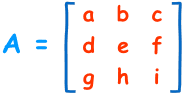The determinant is:

|A| = a(ei − fh) − b(di − fg) + c(dh − eg)
"The determinant of A equals ... etc"

It may look complicated, but there is a pattern: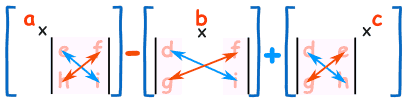To work out the determinant of a 3×3 matrix:

• Multiply a by the determinant of the 2×2 matrix that is not in a's row or column.
• Likewise for b, and for c
• Sum them up, but remember the minus in front of the b

As a formula (remember the vertical bars || mean "determinant of"):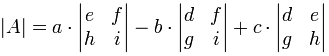"The determinant of A equals a times the determinant of ... etc"

### Example: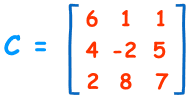|C|= 6×(−2×7 − 5×8) − 1×(4×7 − 5×2) + 1×(4×8 − (−2×2))
= 6×(−54) − 1×(18) + 1×(36)
= −306

## For 4×4 Matrices and Higher

The pattern continues for 4×4 matrices:

• plus a times the determinant of the matrix that is not in a's row or column,
• minus b times the determinant of the matrix that is not in b's row or column,
• plus c times the determinant of the matrix that is not in c's row or column,
• minus d times the determinant of the matrix that is not in d's row or column,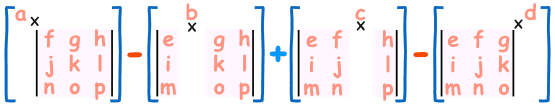As a formula: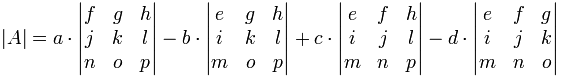Notice the +−+− pattern (+a... b... +c... d...). This is important to remember.

The pattern continues for 5×5 matrices and higher. Usually best to use a Matrix Calculator for those!

## Not The Only Way

This method of calculation is called the "Laplace expansion" and I like it because the pattern is easy to remember. But there are other methods (just so you know).

## Summary

• For a 2×2 matrix the determinant is ad - bc
• For a 3×3 matrix multiply a by the determinant of the 2×2 matrix that is not in a's row or column, likewise for b and c, but remember that b has a negative sign!
• The pattern continues for larger matrices: multiply a by the determinant of the matrix that is not in a's row or column, continue like this across the whole row, but remember the + − + − pattern.